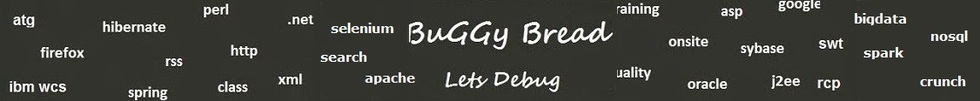## Search Java Classes and Packages

Search Java Frameworks and Libraries

255581 classes and counting ...
 Search Tips Index Status

#Cern.colt.matrix.linalg Classes and Interfaces - 9 results found.
 Name Description Type Package Framework Algebra Linear algebraic matrix operations operating on DoubleMatrix2D; concentrates most functionality of this package. Class cern.colt.matrix.linalg Colt CholeskyDecomposition For a symmetric, positive definite matrix A, the Cholesky decompositionis a lower triangular matrix L so that A = L*L'; Class cern.colt.matrix.linalg Colt EigenvalueDecomposition Eigenvalues and eigenvectors of a real matrix A. Class cern.colt.matrix.linalg Colt LUDecomposition For an m x n matrix A with m >= n, the LU decomposition is an m x nunit lower triangular matrix L, an n x n upper triangular matrix U, Class cern.colt.matrix.linalg Colt LUDecompositionQuick A low level version of LUDecomposition, avoiding unnecessary memory allocation and copying. Class cern.colt.matrix.linalg Colt Matrix2DMatrix2DFunction Interface that represents a function object: a function that takes two arguments and returns a single value. Interface cern.colt.matrix.linalg Colt Property Tests matrices for linear algebraic properties (equality, tridiagonality, symmetry, singularity, etc). Class cern.colt.matrix.linalg Colt QRDecomposition For an m x n matrix A with m >= n, the QR decomposition is an m x northogonal matrix Q and an n x n upper triangular matrix R so that Class cern.colt.matrix.linalg Colt SingularValueDecomposition For an m x n matrix A with m >= n, the singular value decomposition isan m x n orthogonal matrix U, an n x n diagonal matrix S, and Class cern.colt.matrix.linalg Colt